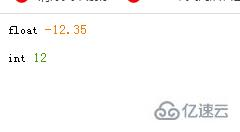# php如何把负数转为正整数

php把负数转为正整数的方法：1、使用abs()函数将负数转为正数，使用intval()函数对正数取整，转为正整数，语法“intval(abs(\$number))”；2、利用“~”位运算符将负数取反加一，语法“~\$number + 1”。php把负数转为正整数的方法

• abs()函数将负数转为正数

• intval()函数对正数取整，转为正整数

```<?php
\$number=-12.35;
var_dump(\$number);
\$result =intval(abs(\$number));
var_dump(\$result);
?>``````<?php
function plus_minus_conversion(\$number = 0){
echo ~\$number + 1;
}
echo plus_minus_conversion(-100.58);
?>```